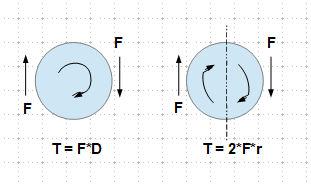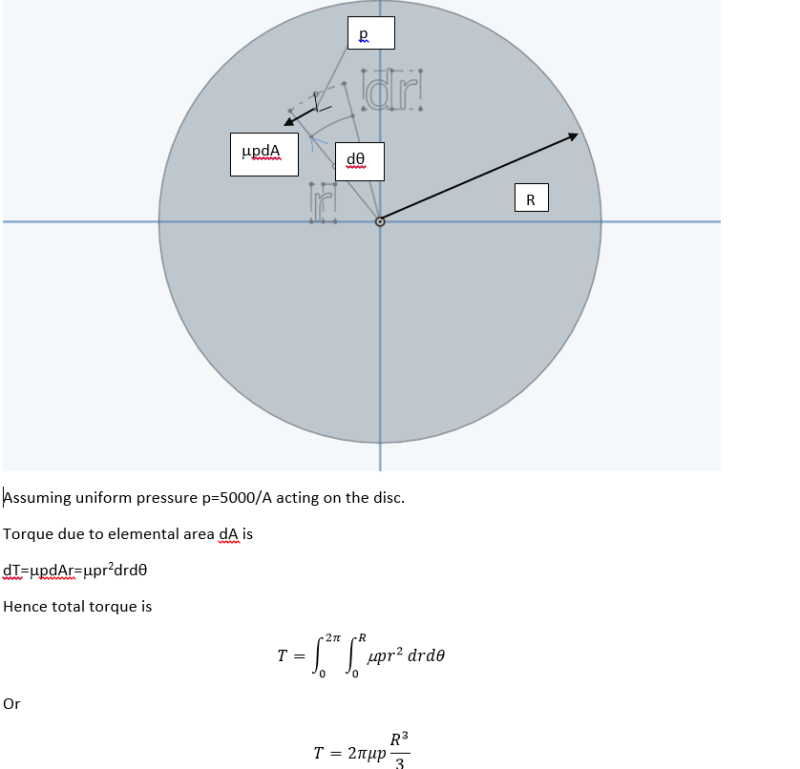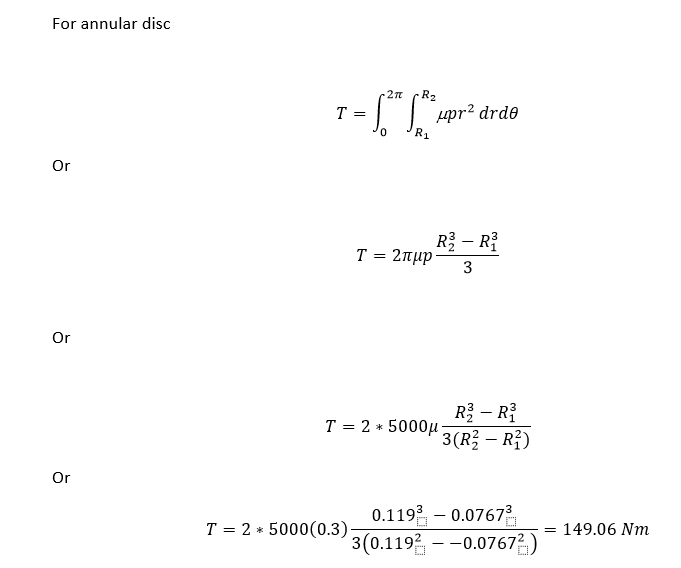×
INTELLIGENT WORK FORUMS
FOR ENGINEERING PROFESSIONALS

Are you an
Engineering professional?
Join Eng-Tips Forums!
• Talk With Other Members
• Be Notified Of Responses
• Keyword Search
Favorite Forums
• Automated Signatures
• Best Of All, It's Free!

*Eng-Tips's functionality depends on members receiving e-mail. By joining you are opting in to receive e-mail.

#### Posting Guidelines

Promoting, selling, recruiting, coursework and thesis posting is forbidden.

# Why bolt torque is T = (Friction factor) x F x D and not the radius?2

## Why bolt torque is T = (Friction factor) x F x D and not the radius?

(OP)
We know that the torque is Force x moment arm. Here in this case why the moment arm is not radius. The real problem for me is that, I am calculating friction torque required by the rotating disc to overcome.
Here the I have done the calculations with below 2 methods:

1. In close approximation with Bolt torque :

Its a plain disc in Martensitic SS rotating on the fixed Bronze plate and acting as a bearing. The axial load is 500 kg including weight of the rotor. Hence like in case of bolt torque, we can say, the Axial force = 500 x 10 = 5000 N ( assuming g = 10 m/s2).
Now, T = 0.3 x 5000 x Avg. dia of the disc ( ( Inner dia. + Outer dia.)/2)

T = 0.3 x 5000 x ((0.119+0.0767)/2)
= 146.77 N-m
2. Considering simple approximation,

T = F x r = 0.3*5000*Avg. radius
= 73.38 N-m

So which one is correct? 73 or 146 Nm of torque?

### RE: Why bolt torque is T = (Friction factor) x F x D and not the radius?

Hi Abhijeet242

watch the first part of this video it covers your rotating disc problem https://www.youtube.com/watch?v=UX-2gwiLzFQ

Further more its much easier to watch the above than me type it all out.

“Do not worry about your problems with mathematics, I assure you mine are far greater.” Albert Einstein

### RE: Why bolt torque is T = (Friction factor) x F x D and not the radius?

The total frictional force is proportional to the contact area or pi*Deff.

### RE: Why bolt torque is T = (Friction factor) x F x D and not the radius?

Area = pi*Deff*(width of contact)

### RE: Why bolt torque is T = (Friction factor) x F x D and not the radius?

"The axial load is 500 kg including weight of the rotor." ... is that kg mass or weight (like a bag of sugar) ?

the difference numerically is moot, but the difference in understanding is significant.

I think you have 500kgf weight, and 1kgf = 10N (near enough) ... you're changing the unit of force, as opposed to determining force from mass.

Can you please explain what it is you're doing ? "bolt torque" is usually the installation torque, to develop a preload. How do you go from weight to torque ??

### RE: Why bolt torque is T = (Friction factor) x F x D and not the radius?

Hi Abhijeet242

The bolt torque calculation using the bolt diameter is an empirical formula obtained from observations and practical tests rather than a theoretical proof.
If you look in any mechanics book and look for lead screws and stuff you will see they use the mean radius for the friction torque.

“Do not worry about your problems with mathematics, I assure you mine are far greater.” Albert Einstein

### RE: Why bolt torque is T = (Friction factor) x F x D and not the radius?

Abhijeet242,

When I am analysing a bolt or screw, I know the diameter, not the radius.

--
JHG

### RE: Why bolt torque is T = (Friction factor) x F x D and not the radius?

What drawoh said, you buy bolts sized on the diameter, and/or measure them by checking the diameter with a pair of calipers. If you need the radius, divide by two.

### RE: Why bolt torque is T = (Friction factor) x F x D and not the radius?

#### Quote (btrueblood)

What drawoh said, you buy bolts sized on the diameter, and/or measure them by checking the diameter with a pair of calipers. If you need the radius, divide by two.

I don't want to divide by two!--
JHG

### RE: Why bolt torque is T = (Friction factor) x F x D and not the radius?

@Desertfox is right. In machine design you analyse a thread, derive the tangential force(P) developed due to axial forces and resulting reaction and frictional forces.

The torque is

T=Pr

### RE: Why bolt torque is T = (Friction factor) x F x D and not the radius?

if that's what yu want to calculate. But the OP is taking the load applied (500kg = 5kN) and trying to find a torque. I don't see what he's trying to accomplish.

### RE: Why bolt torque is T = (Friction factor) x F x D and not the radius?

Torque is related to force couple as shown.### RE: Why bolt torque is T = (Friction factor) x F x D and not the radius?

I think your case is different from screw thread case. Refer derivation below:### RE: Why bolt torque is T = (Friction factor) x F x D and not the radius?

#### Quote (OP)

...Now, T = 0.3 x 5000 x Avg. dia of the disc...

T = 5000µ(2R/3) = 0.333*5000*µ*(2R)= 0.333*5000*µ*D

### RE: Why bolt torque is T = (Friction factor) x F x D and not the radius?

Further to my post above where I have considered circular disk, I have refined the derivation for annular disk (which may be the case with you). The coefficient of friction is 0.3, assumed.It appears that your calculation is approximately true.

### RE: Why bolt torque is T = (Friction factor) x F x D and not the radius?

I only know diameter of a bolt or a screw when analyzing it, and not radius.

### RE: Why bolt torque is T = (Friction factor) x F x D and not the radius?

"I am calculating friction torque required by the rotating disc" where's the screw thread ?

what's weight got to do with a spinning disc ? the MMoI of the disc would be more relevant.

### RE: Why bolt torque is T = (Friction factor) x F x D and not the radius?

Hi rb1957

The OP who doesn’t seem to be responding of late, was confused by the fact that finding the bolt torque involved the bolt diameter and not bolt radius, whereas on this friction disc he should be using 2/3 R for the disc radius, which falls in line with the video link I posted and at the end of this post there is a link to the notes which are from the video link.
The mass of the disc is required I believe, so that he can calculate the frictional torque can be calculated.

http://mechanicsmap.psu.edu/websites/6_friction/di...

“Do not worry about your problems with mathematics, I assure you mine are far greater.” Albert Einstein

### RE: Why bolt torque is T = (Friction factor) x F x D and not the radius?

mass/weight required if that's the normal load, P.

If someone's pushed down on the disc, then it'll be higher.

So this is the torque that needs to be applied to the disc to overcome static friction ... and then it'll start spinning and then MMoI will be important.

### RE: Why bolt torque is T = (Friction factor) x F x D and not the radius?

Yes rb1957 I think at this stage he just wants the required friction torque.

“Do not worry about your problems with mathematics, I assure you mine are far greater.” Albert Einstein

### RE: Why bolt torque is T = (Friction factor) x F x D and not the radius?

confusing to title the thread with something like the bolt preload equation ?

### RE: Why bolt torque is T = (Friction factor) x F x D and not the radius?

(OP)
Dear community, thanks for the overwhelming response. Sorry for the late reply. I see people asking about why am i confusing the torque required to rotate the disc with the Bolt torque.
Well, answer from my point of view, is that, both of the things are accomplishing quiet same results only the difference is that, in case of the Bolt, there is the axial elongation force involved and in case of rotating disc, weight of the rotor is involved. I think answer 74 N-m shall be correct for the specific query. Thanks again for the replies.

Now, speaking about the bolt, what i understood is that, Torque universally will always be T = F x r. In case of bolt now, previously what i was missing is, there are 2 surface friction bolt and nut experiences.
2. Friction between the Nut bottom surface and the surface of the component on which it is being tightened.
Here, the K factor which we use while calculating tightening torque is actually considering both these frictions and during the derivation "/2" has been taken care (i.e. r = d/2). Hence the simplified form what we see is T = K.F.d
where, d = bolt diameter.

### RE: Why bolt torque is T = (Friction factor) x F x D and not the radius?

I think you've found the help you needed but I'm curious, where did you see T = KFd? If you are using a nut and the nut is not spinning then there would not be a friction torque between it and the object it is tightening against.

Usually there are two components causing friction torque - friction between bolt head and surface and friction between bolt threads and mating part.

Maybe the source that supplied the T = KFd formula combined the two. That's quite the simplification though. At any rate, the numbers are all washed out by the choice of K.

#### Red Flag This Post

Please let us know here why this post is inappropriate. Reasons such as off-topic, duplicates, flames, illegal, vulgar, or students posting their homework.

#### Red Flag Submitted

Thank you for helping keep Eng-Tips Forums free from inappropriate posts.
The Eng-Tips staff will check this out and take appropriate action.

#### Resources

Low-Volume Rapid Injection Molding With 3D Printed Molds
Learn methods and guidelines for using stereolithography (SLA) 3D printed molds in the injection molding process to lower costs and lead time. Discover how this hybrid manufacturing process enables on-demand mold fabrication to quickly produce small batches of thermoplastic parts. Download Now
Examine how the principles of DfAM upend many of the long-standing rules around manufacturability - allowing engineers and designers to place a partâ€™s function at the center of their design considerations. Download Now
Industry Perspective: Education and Metal 3D Printing
Metal 3D printing has rapidly emerged as a key technology in modern design and manufacturing, so itâ€™s critical educational institutions include it in their curricula to avoid leaving students at a disadvantage as they enter the workforce. Download Now

Close Box

# Join Eng-Tips® Today!

Join your peers on the Internet's largest technical engineering professional community.
It's easy to join and it's free.

Here's Why Members Love Eng-Tips Forums:

•Talk To Other Members
• Notification Of Responses To Questions
• Favorite Forums One Click Access
• Keyword Search Of All Posts, And More...

Register now while it's still free!